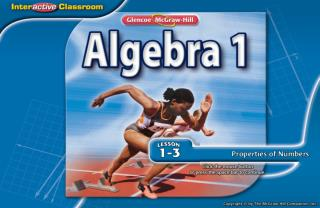DownloadDownload PresentationSplash Screen

# Splash Screen

Télécharger la présentation## Splash Screen

- - - - - - - - - - - - - - - - - - - - - - - - - - - E N D - - - - - - - - - - - - - - - - - - - - - - - - - - -
##### Presentation Transcript

1. Splash Screen

2. Five-Minute Check (over Lesson 1–2) Then/Now New Vocabulary Key Concept: Properties of Equality Key Concept: Addition Properties Key Concept: Multiplication Properties Example 1: Evaluate Using Properties Key Concept: Commutative Property Key Concept: Associative Property Real-World Example 2: Apply Properties of Numbers Example 3: Use Multiplication Properties Lesson Menu

3. You used the order of operations to simplify expressions. (Lesson 1-2) • Recognize the properties of equality and identity. • Recognize the Commutative and Associative Properties. Then/Now

4. equivalent expressions • additive identity • multiplicative identity • multiplicative inverse • reciprocal Vocabulary

5. KC 1

6. KC 2

7. KC 3

8. Name the property used in each step. Evaluate Using Properties Substitution; 12 – 8 = 4 Substitution; 15 ÷ 5 = 3 Substitution; 3 – 2 = 1 Example 1

9. Multiplicative Inverse; ● 4 = 1 Evaluate Using Properties Multiplicative Identity; 3 ● 1 = 3 = 4 Substitution; 1 + 3 = 4 Answer: 4 Example 1

10. 4 • 5 • 1 • 0 [Enter question here]

11. KC 4

12. KC 5

13. 4.25 + 6.50 + 9.00 + 7.75 Apply Properties of Numbers HORSEBACK RIDINGMigina made a list of trail lengths to find the total miles she rode. Find the total miles Migina rode her horse. Bent Tree Knob Hill Meadowrun Pinehurst Example 2

14. Apply Properties of Numbers = 4.25 + 7.75 + 6.50 + 9.00 Commutative (+) = (4.25 + 7.75) + (6.50 + 9.00) Associative (+) = 12.00 + 15.50Substitution =27.50Substitution Answer: Migina rode 27.5 miles on the trails. Example 2

15. TRANSPORTATION Darlene rode the city train from the Winchester Street Station to the airport. How far did she travel on the train? • 4.5 mi • 5.5 mi • 6.0 mi • 6.2 mi [Enter question here]

16. Use Multiplication Properties Evaluate 2 ● 8 ● 5 ● 7 using properties of numbers. Name the property used in each step. You can rearrange and group the factors to make mental calculations easier. 2 ● 8 ● 5 ● 7 = 2 ● 5 ● 8 ● 7 Commutative (×) = (2 ● 5) ● (8 ● 7) Associative (×) = 10 ● 56 Multiply mentally. = 560 Multiply mentally. Answer: 560 Example 3

17. Evaluate 3 ● 5 ● 3 ● 4. • 45 • 36 • 15 • 180 [Enter question here]

18. End of the Lesson

19. A B C D A. 4 B. 5 C. 1 D. 0 Example 1

20. A B C D TRANSPORTATION Darlene rode the city train from the Winchester Street Station to the airport. How far did she travel on the train? A. 4.5 mi B. 5.5 mi C. 6.0 mi D. 6.2 mi Example 2

21. A B C D Evaluate 3 ● 5 ● 3 ● 4. A. 45 B. 36 C. 15 D. 180 Example 3